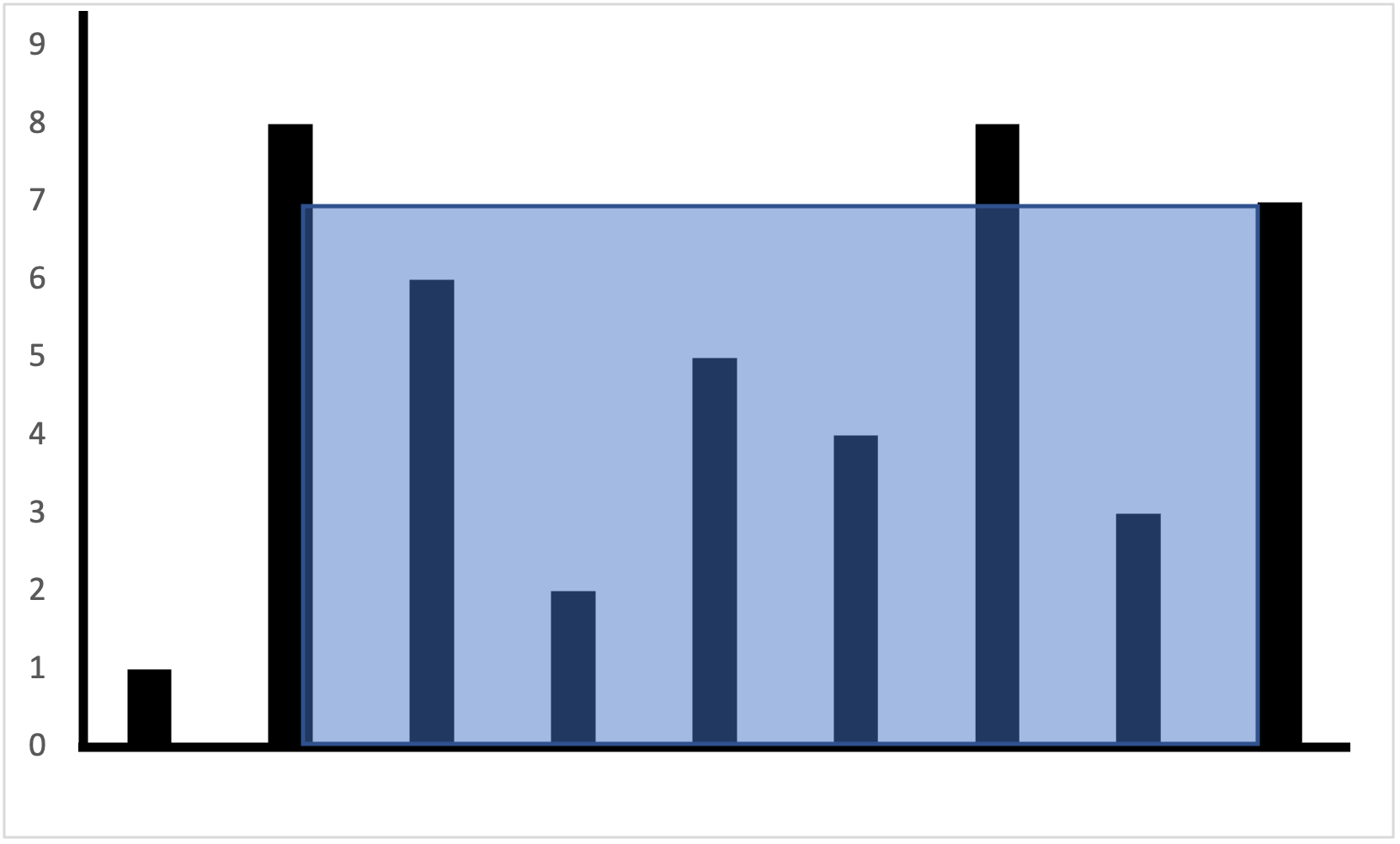Career coaching for women in tech.

# Leetcode: Container With Most Water

I love solving Leetcode coding problems for fun. I came across this problem and was intrigued to prove why the sliding window algorithm is correct.

Given an array of heights, find two lines that together with the x-axis form a container, such that the container contains the most water.Representation of [1,8,6,2,5,4,8,3,7]. For this input, the most water a container can contain is 49.

You can solve this brute force by comparing all possible combinations of left and right lines and keeping track of the most water. The complexity of this solution is quadratic.

``````function maxWaterQuadratic(height) {
let left = 0;
let right = 1;
let max = 0;

while(right < height.length) {
while(left < right) {
let h = Math.min(height[right], height[left]);
max = Math.max(max, h*(right - left));
left++;
}
right++;
left = 0;
}

return max;
};
``````

Algorithms with quadratic complexity do not work well for very large datasets. For example, given an array with 100 entries as input to a quadratic algorithm takes on the order of `100^2 = 10,000` instructions. No problem for a modern computer. But as the array size increases, say to 300 million (population size of the US), now we need something on the order of `90,000,000,000,000,000` instructions. Given that we measure CPUs in GHz (billions of instructions), a quadratic algorithm would not work for this scale. In fact, if you submit this algorithm on Leetcode, you get a Time Limit Exceeded error for one of the test cases.

## Linear vs quadratic complexity

Can we solves this problem with linear complexity? Is there an algorithm that looks at each array entry just once (or a constant multiple of once) instead of all combinations of pairs of entries?

In fact, yes, there's a solution that runs in linear time: Start with the widest container. That means use the first and last element as the left and right boundary. Move the shorter of the left and right boundary one step inwards. Keep track of the most water until the left and right boundaries overlap. This is a sliding window algorithm where one pointer starts at the front, the other at the back.

``````function maxWaterLinear(height) {
let max = 0;
let left = 0;
let right = height.length-1;
while(left < right) {
let area = (right - left) * Math.min(height[left], height[right]);
max = Math.max(area, max);
height[left] < height[right]) ? left++ : right--;
}

return max;
};
``````

Why is this algorithm correct though? It passes all the test cases on Leetcode - that doesn't prove correctness though. How do we know there isn't some edge case where this algorithm would give us the wrong solution?

## Proof of Sliding Window Algorithm

Suppose the real solution of the problem is from index `a` to `b` with water height `h.` Then both `a` and `b` are greater or equal to `h`. Then for any index to the left or to the right of the optimal container, the height must be less than `h`. Otherwise we could extend the optimal container to that index without sacrificing height and have a larger water container.

In our sliding window algorithm, as we move our indexes from the outside in, we will eventually reach `a` or `b`. Suppose we reach `a` first. We just proved that everything outside of the other index `b`, must be smaller than `h` and thus smaller than `a`. Therefore, one pointer in our algorithm will keep moving until it reaches `b` while the other pointer stays on `a.` At which point the optimal volume is recorded. If we reach `b` first, the argument is exactly the same. Thus, this linear time algorithm will always find the optimal solution.

Happy algorithm coding!

Subscribe to the newsletter
I'll only send emails when new content is posted. No spam.